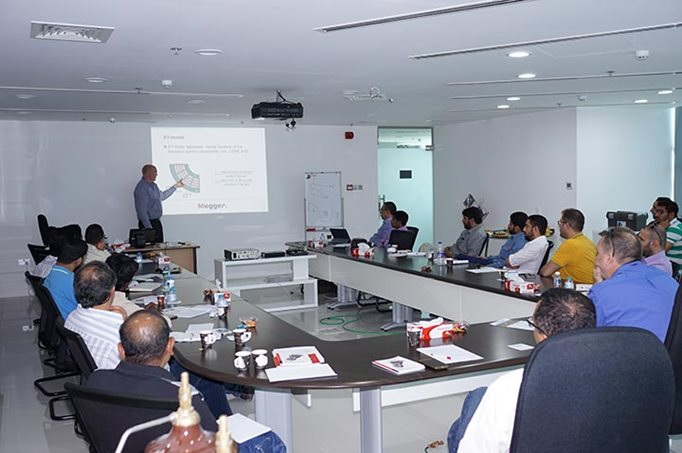Company
News
Transformer Training Course

# Transformer Training CourseDay1 (Introduction day):

• Theoretical presentation. ( introduction to transformer, various test methods, excitation current measurement, ratio and winding resistance, etc)

• Practical session ( basic tests, ratio test, winding resistance, leakage reactance, excitation current using TTR , MTO and TRAX)

Day2 (Insulation diagnostics day):

• Theoretical presentation. ( introduction to insulation testing, AC vs DC,Delta4000, DFR, ITC, IDAX, introduction to bushing test)

• Practical session ( Delta4000, IDAX, bushing test using tip up,)

Day3 (Advanced testing day):

• Theoretical presentation ( SFRA basics, introduction to FRAX, introduction to OLTC and DRM measurement, FRSL tests) 12pm to 1 pm break

• Practical session ( FRAX, TRAX, final Q/A session)